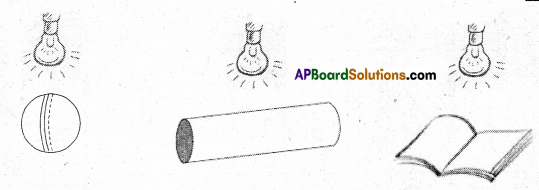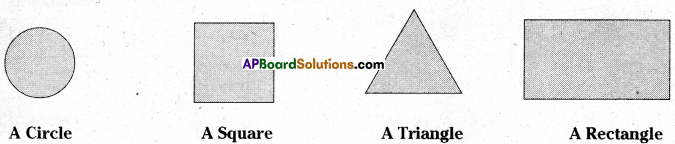AP State Syllabus AP Board 7th Class Maths Solutions Chapter 14 Understanding 3D and 2D Shapes Ex 4 Textbook Questions and Answers.

## AP State Syllabus 7th Class Maths Solutions 14th Lesson Understanding 3D and 2D Shapes Exercise 4Question 1.
A bulb is kept burning just right above the following solids. Name the shape of the shadows obtained in each case. Attempt to give a rough sketch of the shadow. (You may try to experiment first and then answer these questions).Solution:
A ball -Circle
A cylindrical pipe – Rectangle
A book – RectangleQuestion 2.
Here are the shadows of some 3D objects, when seen under the lamp of an overhead projector. Identify the solid(s) that match each shadow. (There may be many answers for these!)Solution:
i) A Circle – Spherical/circular objects
ii) A Square – Cube/square sheets
iii) A Triangle – Triangular/right prism with triangular base
iv) A Rectangle – Cuboid/rectangular shapes.